# Test: Square Root And Cube Root- 2

## 10 Questions MCQ Test Quantitative Aptitude for GMAT | Test: Square Root And Cube Root- 2

Description
Attempt Test: Square Root And Cube Root- 2 | 10 questions in 20 minutes | Mock test for Banking Exams preparation | Free important questions MCQ to study Quantitative Aptitude for GMAT for Banking Exams Exam | Download free PDF with solutions
QUESTION: 1

### Practice Quiz or MCQ (Multiple Choice Questions) with solutions are available for Practice, which would help you prepare for "Square Root and Cube Root" under Quantitative Aptitude. You can practice these practice quizzes as per your speed and improvise the topic. The same topic is covered under various competitive examinations like - CAT, GMAT, Bank PO, SSC and other competitive examinations.   Q. The cube root of .000216 is:

Solution: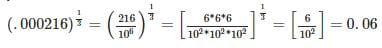QUESTION: 2

### What should come in place of both x in the equation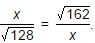Solution: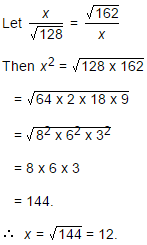QUESTION: 3

### The least perfect square, which is divisible by each of 21, 36 and 66 is:

Solution:

L.C.M. of 21, 36, 66 = 2772.

Now, 2772 = 2 x 2 x 3 x 3 x 7 x 11

To make it a perfect square, it must be multiplied by 7 x 11.

So, required number = 22 x 32 x 72 x 112 = 213444

QUESTION: 4

What is the Square Root of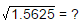Solution: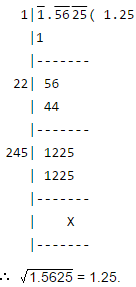QUESTION: 5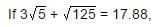then what will be the value of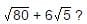Solution: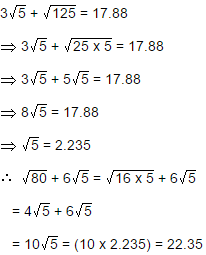QUESTION: 6

If a = 0.1039, then the value of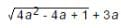is:

Solution:
QUESTION: 7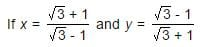then the value of (x2 + y2) is:

Solution: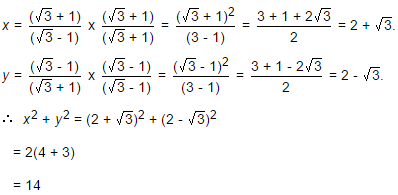QUESTION: 8

A group of students decided to collect as many paise from each member of group as is the number of members. If the total collection amounts to Rs. 59.29, the number of the member is the group is:

Solution: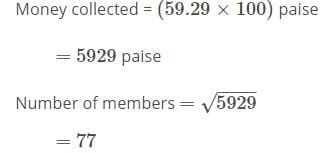QUESTION: 9

The square root of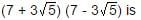Solution: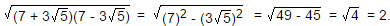QUESTION: 10

If √5 = 2.236, then the value of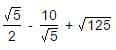is equal to:

Solution: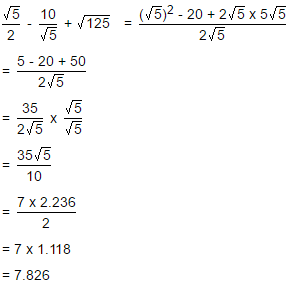Use Code STAYHOME200 and get INR 200 additional OFF Use Coupon Code